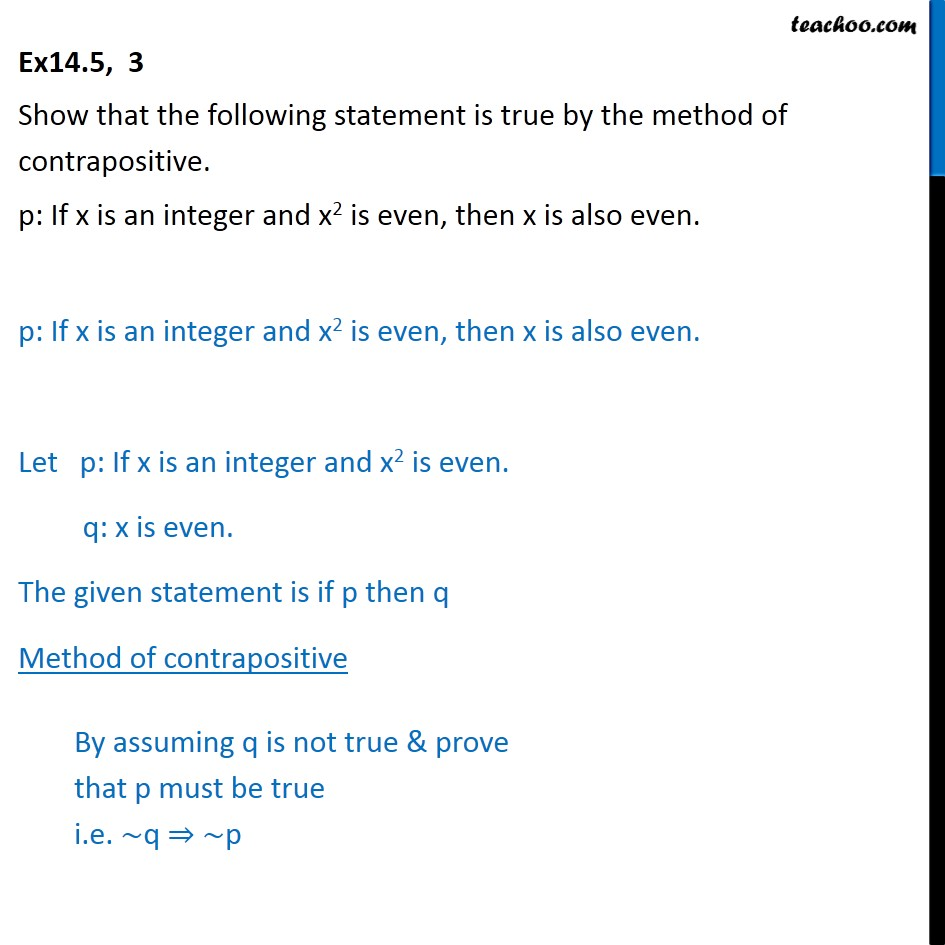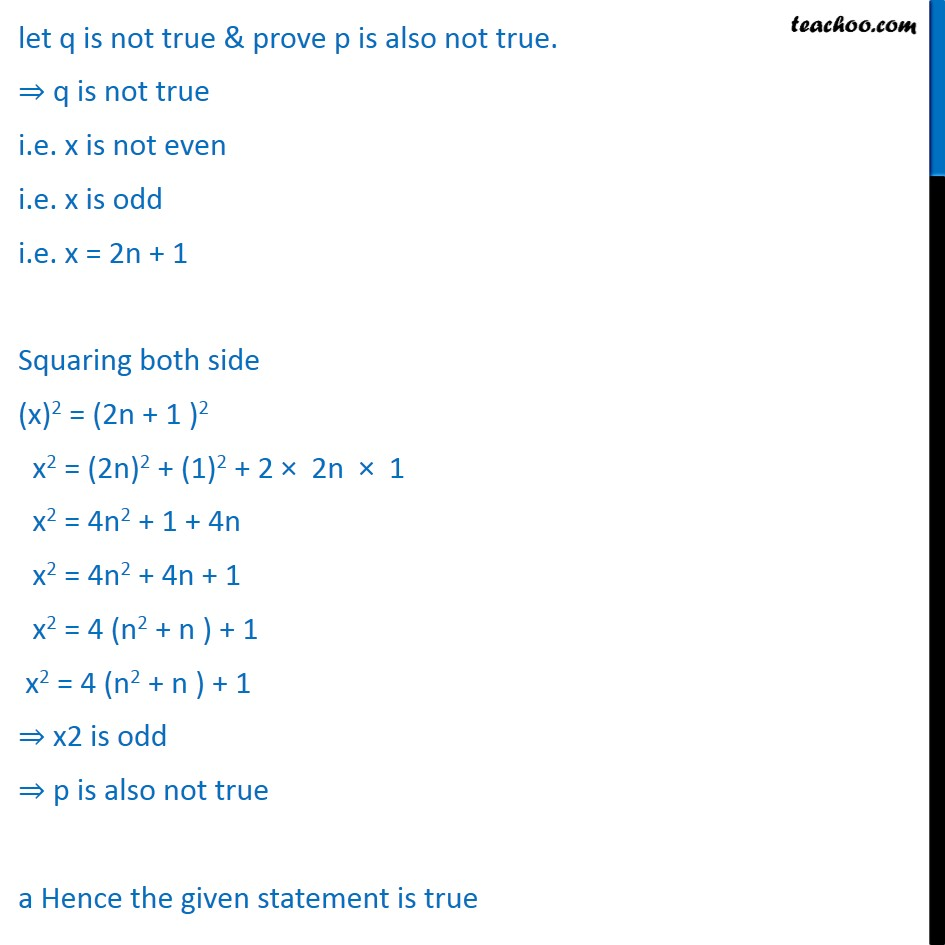Ex 14.5

Chapter 14 Class 11 Mathematical Reasoning
Serial order wiseGet live Maths 1-on-1 Classs - Class 6 to 12

### Transcript

Ex 14.5, 3 Show that the following statement is true by the method of contrapositive. p: If x is an integer and x2 is even, then x is also even. p: If x is an integer and x2 is even, then x is also even. Let p: If x is an integer and x2 is even. q: x is even. The given statement is if p then q Method of contrapositive let q is not true & prove p is also not true. q is not true i.e. x is not even i.e. x is odd i.e. x = 2n + 1 Squaring both side (x)2 = (2n + 1 )2 x2 = (2n)2 + (1)2 + 2 2n 1 x2 = 4n2 + 1 + 4n x2 = 4n2 + 4n + 1 x2 = 4 (n2 + n ) + 1 x2 = 4 (n2 + n ) + 1 x2 is odd p is also not true a Hence the given statement is true